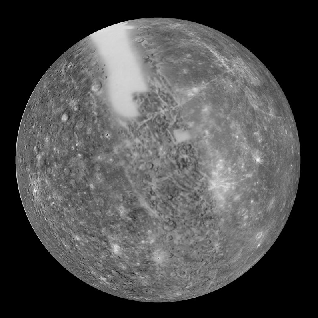﻿ Weight On Mercury Calculator ﻿# Weight on Mercury Calculator### How Much Would You Or Any Object Weigh On Mercury?Enter Weight: Any unit grams (g) kilograms (kg) pounds (lbs)

This Weight on Mercury Conversion Calculator calculates your weight or the weight that any object would be if on Mercury.

To use this calculator, a user just enters his or her weight or the object's weight and clicks the 'Calculate' button, and the weight will automatically be computed and shown below. The weight can be entered in any units and the resultant answer will be in the same units which the user has input. Thus, for example, if a user enters 330lbs, the resultant answer of the weight in Mercury would be 124.46lbs. The units will always match. Just for the sake of clarity, a user can select the units he wants the answer to appear in, whether grams, kilograms, or pounds, and that unit will show in the answer.

This calculator calculates the weight on Mercury from the weight of the object on earth. Being that it takes the weight of an object on earth and converts it to the weight on Mercury, the formula is Weight on Mercury= (Weight on Earth/9.81m/s2) * 3.7m/s2. To find the weight on Mercury, we divide the weight on earth by the earth's force of gravity, which is 9.81m/s2. This calculates the mass of the object. Once we have the object's mass, we can find the weight by multiplying it by the gravitational force, which it is subject to. Being that Mercury has a gravitational force of 8.87m/s2, we multiply the object's mass by this quanitity to calculate an object's weight on Mercury.

So an object or person on Mercury would weigh 37.72% its weight on earth. Therefore, a person would be lighter on Mercury than on earth. Conversely, a person is 62.28%% heavier on earth than on Mercury.

What causes the differences in weight between the various planets? The answer is the gravitational force which a planet is subject to. Earth has much more mass than Mercury. In fact, Mercury is the smallest planet. Therefore, the sun pulls on the earth with greater force, because of the greater mass. You probably are familiar that gravity is a downward force. It pushes you back to the Earth's surface. This is why when you jump, you come back down. Being that the earth has greater gravitational force, it pulls object downward with greater force. This translates into greater weight.

If you want to calculate the weight of all of the planets simultaneously, see our Weight on Planets Calculator.

Related Resources

﻿